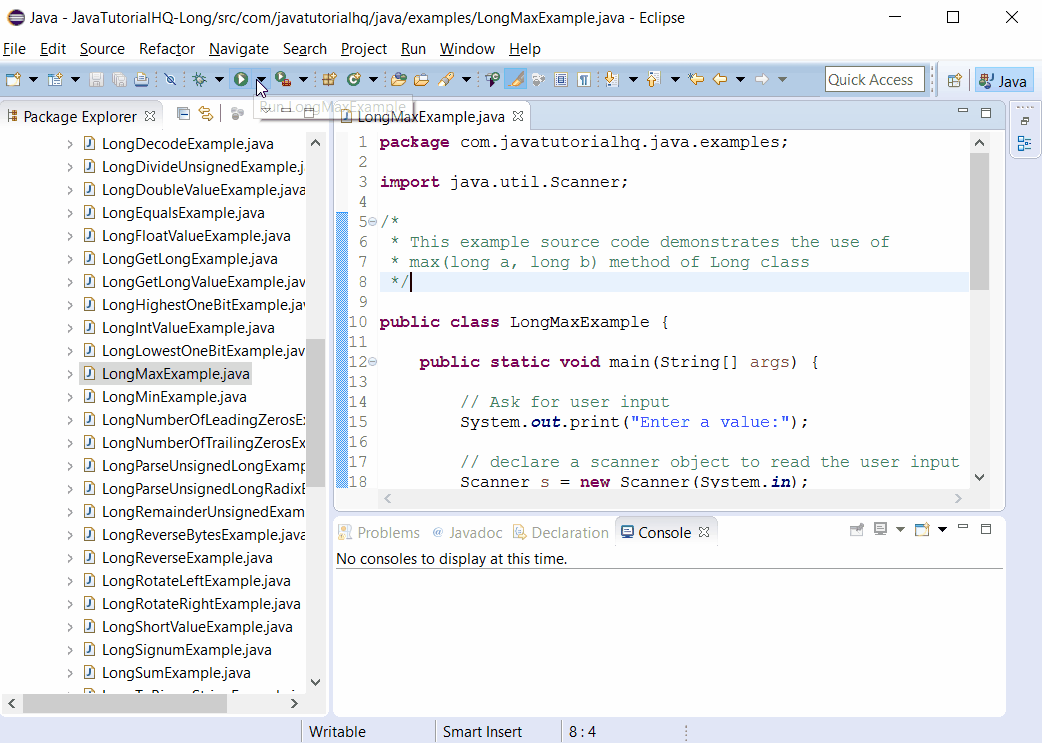Java Long max(long a, long b) method example

HQ » Java Tutorial » java.lang » Long » max(long a, long b)
Java Long max(long a, long b) method example 2018-02-16T07:26:13+00:00

Description

The Long.max(long a, long b) java method returns the greater of two long values as if by calling Math.max.

Make a note that the max() method of Long class is static thus it should be accessed statically which means the we would be calling this method in this format:

Long.max(method args)

Non static method is usually called by just declaring method_name(argument) however in this case since the method is static, it should be called by appending the class name as suffix. We will be encountering a compilation problem if we call the java max method non statically.

Method Syntax

public static long max(long a, long b)

Method Argument

Data Type Parameter Description
long a the first operand
long b the second operand

Method Returns

The max(long a, long b) method of Long class returns the greater of a and b.

Compatibility

Requires Java 1.8 and up

Java Long max(long a, long b) Example

Below is a simple java example on the usage of max(long a, long b) method of Long class.

Sample Output

Below is the sample output when you run the above example.References

Singapore

Our Goal

JavaTutorialHQ aims to to be The Ultimate Guide on Java with hundreds of examples from basic to advance Topics.